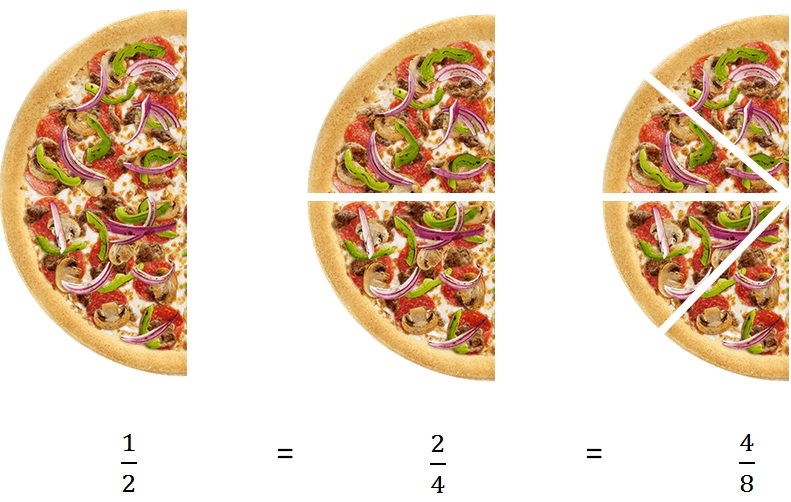Equivalent Rational Numbers

Chapter 9 Class 7 Rational Numbers
Concept wise

Equivalent rational numbers are same as equivalent fractions.

Suppose as rational number isHere

1/2 = 2/4 = 4/6

So, these are equivalent rational numbers

Similarly,

we can have negative numbers as well

(-1)/2 = (-2)/4 = (-4)/8

Now,

How to find these equivalent rational numbers?

Get live Maths 1-on-1 Classs - Class 6 to 12